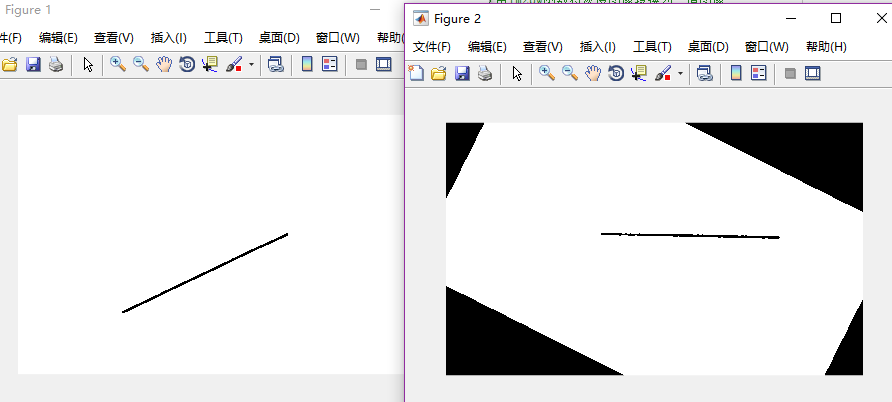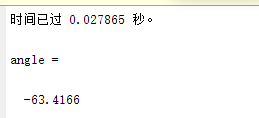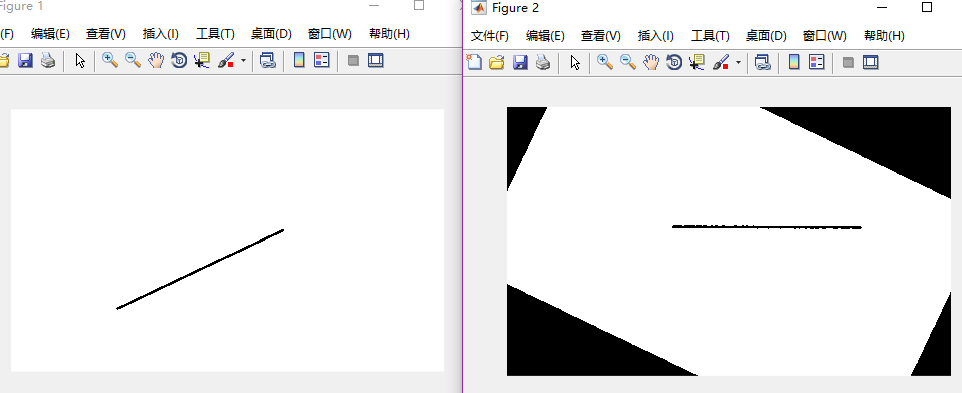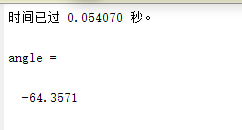# 文档图像倾斜角检测及校正（三）

• 斜率空间投票原理
• Matlab程序

### 阅读之前注意：

Hi，你好，我是Cooper Liu，欢迎来到我写的“文档图像校正”系列博客。基于三种原理，我写了四个实验性的Matlab验证程序，以及两个文档校正Matlab程序。在这里你将能够获取所有的源代码以及测试图片，完全可以在你自己的Matlab上跑这些程序。

Matlab程序2

## Matlab程序%%本版基于斜率空间投票原理
%%2018.01.20_14:09 by Cooper Liu
%%Questions? Contact me: angelpoint@foxmail.com
clear;clc; %清空之前的变量
level=graythresh(I); %使用最大类间方差法找到图片的一个合适的阈值
bw=im2bw(I,level); %根据阈值，使用im2bw函数将灰度图像转换为二值图像
figure(1);imshow(bw);
[m,n]=size(bw); %获取尺寸
flag=0;
tic; %计时开始
for i=1:m
for j=1:n
if bw(i,j)==0
if flag==0
x0=i;y0=j; %获取第一个点
flag=1;
end
end
end
end
range=10000; %range越大对角度分得更细，计算量更大
low=-1;
high=3;

a=zeros(range,1);
for i=1:m
for j=1:n
if bw(i,j)==0
if i==x0
k=2;
area=floor((k-(-1))/4*range); %向下取整
a(area+1,1)=a(area+1,1)+1; %区间计数
else
k=(j-y0)/(i-x0);
k2=k;
if  k>1 || k<-1
k2=2-1/k; %转换斜率，保证斜率范围（-1, 3）
end
area=floor((k2-(-1))/4*range); %向下取整
a(area+1,1)=a(area+1,1)+1;
end
end
end
end
toc %获取时间
[s_i,index] = sort(a,'descend'); %降序排序并获取原来序列的序号至index
max=index(1);
tmp=(-1)+max/range*4;
if tmp>1
k=1/(2-tmp); %还原原来的斜率
else
k=tmp;
end
angle=atan(k); %用atan求出来的角度在-pi/2到+pi/2之间
angle=angle*180/pi

rot=90-angle;
pic=imrotate(I,rot,'crop'); %旋转图像
figure(2);imshow(pic);


%%本版基于斜率空间投票原理，改进自angleDetection3.m
%%2018.01.20_15:41 by Cooper Liu
%%Questions? Contact me: angelpoint@foxmail.com
clear;clc; %清空之前的变量
level=graythresh(I); %使用最大类间方差法找到图片的一个合适的阈值
bw=im2bw(I,level); %根据阈值，使用im2bw函数将灰度图像转换为二值图像时
figure(1);imshow(bw);
[m,n]=size(bw); %获取尺寸
flag=0;

for i=1:m
for j=1:n
if bw(i,j)==0
if flag==0
x0=i;y0=j;
flag=1;
end
end
end
end
range=10; %整个斜率区间分为10个子区间
low=-1;
high=3;
tmpLow=low;
tmpHigh=high;
max=0;
tic; %开始计时
while high-low>0.0001
a=zeros(range,1);
for i=1:m
for j=1:n
if bw(i,j)==0
if i==x0 %如果x坐标与x0相同
k=2; %则固定k为2
if k>=low && k<=high
area=floor((k-low)/(high-low)*range); %向下取整
if area==range %如果等于最后一个区间
area=area-1; %请考虑到后面区间计数的area+1
end
a(area+1,1)=a(area+1,1)+1; %区间计数
end
else     %如果x坐标与x0不同
k=(j-y0)/(i-x0); %则计算斜率
k2=k;
if  k>1 || k<-1
k2=2-1/k;
end
if k2>=low && k2<=high
area=floor((k2-low)/(high-low)*range); %向下取整
if area==range
area=area-1;
end
a(area+1,1)=a(area+1,1)+1;
end
end
end
end
end
[s_i,index] = sort(a,'descend');
max = index(1);
tmpLow = low;
tmpHigh = high;
if max>=2 && max<=9
else
end
low = tmpLow + (max - 1 - areaAddition)/range*(tmpHigh - tmpLow);
high = tmpLow + (max + areaAddtion)/range*(tmpHigh - tmpLow);
end

toc %获取计时时间

tmp=tmpLow+max/range*(tmpHigh-tmpLow);
if tmp>1
k=1/(2-tmp);
else
k=tmp;
end
angle=atan(k); %用atan求出来的角度在-pi/2到+pi/2之间
angle=angle*180/pi

rot=90-angle;
pic=imrotate(I,rot,'crop'); %旋转图像
figure(2);imshow(pic);### 文章目录

1. 参考文献： 荆雷，张欣，郭金鑫．基于版面的拍照文档图像倾斜校正.激光与红外[J]．2010，第10期 ↩︎

2. 同时感谢愿意在网络上分享自己想法的各位博主。 ↩︎

05-10
11-0646004-11
06-07
01-232944
01-236231
04-20
01-212万+
03-223550